SNAP Library 6.0, Developer Reference  2020-12-09 16:24:20 SNAP, a general purpose, high performance system for analysis and manipulation of large networks
TSsParser Class Reference

`#include <ss.h>`

Collaboration diagram for TSsParser: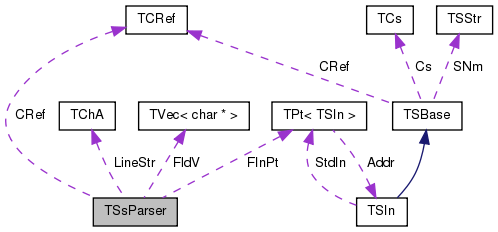[legend]

## Public Member Functions

TSsParser (const TStr &FNm, const TSsFmt _SsFmt=ssfTabSep, const bool &_SkipLeadBlanks=false, const bool &_SkipCmt=true, const bool &_SkipEmptyFld=false)
Constructor. More...

TSsParser (const TStr &FNm, const char &Separator, const bool &_SkipLeadBlanks=false, const bool &_SkipCmt=true, const bool &_SkipEmptyFld=false)
Constructor. More...

~TSsParser ()

bool Next ()
Loads next line from the input file. More...

bool NextSlow ()
Loads next line from the input file (older, slow implementation - deprecated). More...

int Len () const
Returns the number of fields in the current line. More...

int GetFlds () const
Returns the number of fields in the current line. More...

uint64 GetLineNo () const
Returns the line number of the current line. More...

bool IsCmt () const
Checks whether the current line is a comment (starts with '#'). More...

bool Eof () const
Checks for end of file. More...

TChA GetLnStr () const
Returns the current line. More...

void ToLc ()
Transforms the current line to lower case. More...

const char * GetFld (const int &FldN) const
Returns the contents of the field at index `FldN`. More...

char * GetFld (const int &FldN)
Returns the contents of the field at index `FldN`. More...

const char * operator[] (const int &FldN) const
Returns the contents of the field at index `FldN`. More...

char * operator[] (const int &FldN)
Returns the contents of the field at index `FldN`. More...

bool GetInt (const int &FldN, int &Val) const
If the field `FldN` is an integer its value is returned in `Val` and the function returns `true`. More...

int GetInt (const int &FldN) const
Assumes `FldN` is an integer its value is returned. If `FldN` is not an integer an exception is thrown. More...

bool IsInt (const int &FldN) const
Checks whether fields `FldN` is an integer. More...

bool GetFlt (const int &FldN, double &Val) const
If the field `FldN` is a float its value is returned in `Val` and the function returns `true`. More...

bool IsFlt (const int &FldN) const
Checks whether fields `FldN` is a float. More...

double GetFlt (const int &FldN) const
Assumes `FldN` is a floating point number its value is returned. If `FldN` is not an integer an exception is thrown. More...

bool GetUInt64 (const int &FldN, uint64 &Val) const
If the field `FldN` is a 64-bit unsigned integer its value is returned in `Val` and the function returns `true`. More...

bool IsUInt64 (const int &FldN) const
Checks whether fields `FldN` is unsigned 64-bit integer number. More...

uint64 GetUInt64 (const int &FldN) const
Assumes `FldN` is a 64-bit unsigned integer point number its value is returned. If `FldN` is not a 64-bit unsigned integer an exception is thrown. More...

const char * DumpStr () const

## Static Public Member Functions

static PSsParser New (const TStr &FNm, const TSsFmt SsFmt)

## Private Member Functions

UndefDefaultCopyAssign (TSsParser)

## Private Attributes

TCRef CRef

TSsFmt SsFmt
Separator type. More...

Ignore leading whitespace characters in a line. More...

bool SkipCmt
Skip comments (lines starting with #). More...

bool SkipEmptyFld
Skip empty fields (i.e., multiple consecutive separators are considered as one). More...

uint64 LineCnt
Number of processed lines so far. More...

char SplitCh
Separator character (if one of the non-started separators is used) More...

TChA LineStr
Current line. More...

TVec< char * > FldV
Pointers to fields of the current line. More...

PSIn FInPt
Pointer to the input file stream. More...

## Friends

class TPt< TSsParser >

## Detailed Description

Definition at line 72 of file ss.h.

## Constructor & Destructor Documentation

 TSsParser::TSsParser ( const TStr & FNm, const TSsFmt _SsFmt = `ssfTabSep`, const bool & _SkipLeadBlanks = `false`, const bool & _SkipCmt = `true`, const bool & _SkipEmptyFld = `false` )

Constructor.

Parameters
 FNm Input filename. Can be a text file or a compressed file. _SsFmt Spread-sheet separator format. Each line will be broken in a set of fields, where the boundary between the fields is defined by the `_SsFmt`. _SkipLeadBlanks If `true` leading/trailing white-spaces of the line will be ignored. _SkipCmt If `true` lines starting with '#' will be considered as comments and will be skipped. _SkipEmptyFld If `true` then empty fields (consecutive occurrences of the separator) will be ignored.

Definition at line 351 of file ss.cpp.

351  : SsFmt(_SsFmt),
353  if (TZipIn::IsZipExt(FNm.GetFExt())) { FInPt = TZipIn::New(FNm); }
354  else { FInPt = TFIn::New(FNm); }
355  //Bf = new char [BfLen];
356  switch(SsFmt) {
357  case ssfTabSep : SplitCh = '\t'; break;
358  case ssfCommaSep : SplitCh = ','; break;
359  case ssfSemicolonSep : SplitCh = ';'; break;
360  case ssfVBar : SplitCh = '|'; break;
361  case ssfSpaceSep : SplitCh = ' '; break;
362  case ssfWhiteSep: SplitCh = ' '; break;
363  default: FailR("Unknown separator character.");
364  }
365 }
TSsFmt SsFmt
Separator type.
Definition: ss.h:74
uint64 LineCnt
Number of processed lines so far.
Definition: ss.h:78
Semicolon separated.
Definition: ss.h:8
TStr GetFExt() const
Definition: dt.cpp:1421
Vertical bar separated.
Definition: ss.h:9
static PSIn New(const TStr &FNm)
Definition: zipfl.cpp:122
TVec< char * > FldV
Pointers to fields of the current line.
Definition: ss.h:81
static PSIn New(const TStr &FNm)
Definition: fl.cpp:290
TChA LineStr
Current line.
Definition: ss.h:80
Whitespace (space or tab) separated.
Definition: ss.h:11
Tab separated.
Definition: ss.h:6
#define FailR(Reason)
Definition: bd.h:240
Space separated.
Definition: ss.h:10
PSIn FInPt
Pointer to the input file stream.
Definition: ss.h:82
static bool IsZipExt(const TStr &FNmExt)
Check whether the file extension FNmExt is that of a compressed file (.gz, .7z, .rar, .zip, .cab, .arj. bzip2).
Definition: zipfl.cpp:199
bool SkipCmt
Skip comments (lines starting with #).
Definition: ss.h:76
Ignore leading whitespace characters in a line.
Definition: ss.h:75
char SplitCh
Separator character (if one of the non-started separators is used)
Definition: ss.h:79
bool SkipEmptyFld
Skip empty fields (i.e., multiple consecutive separators are considered as one).
Definition: ss.h:77
Comma separated.
Definition: ss.h:7

Here is the call graph for this function: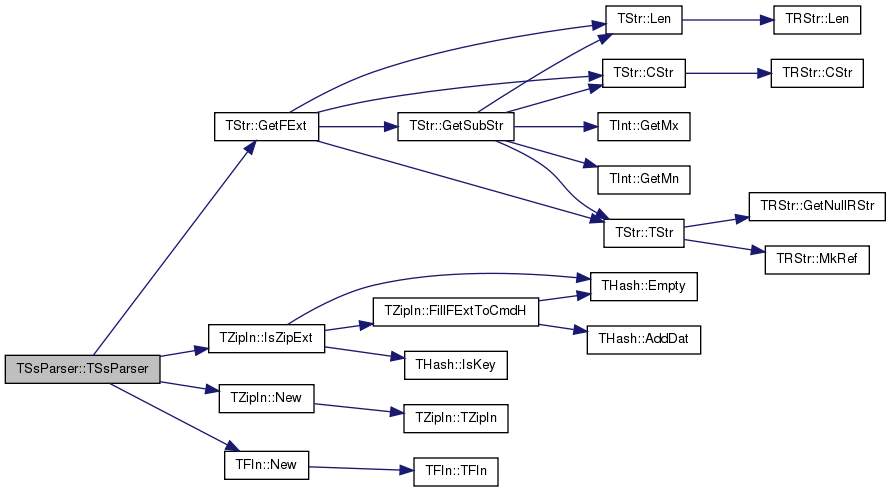TSsParser::TSsParser ( const TStr & FNm, const char & Separator, const bool & _SkipLeadBlanks = `false`, const bool & _SkipCmt = `true`, const bool & _SkipEmptyFld = `false` )

Constructor.

Parameters
 FNm Input filename. Can be a text file or a compressed file. Separator Spread-sheet separator character. Each line will be broken in a set of fields, where the boundary between the fields is the `Separator` character. _SkipLeadBlanks If `true` leading/trailing white-spaces of the line will be ignored. _SkipCmt If `true` lines starting with '#' will be considered as comments and will be skipped. _SkipEmptyFld If `true` then empty fields (consecutive occurrences of the separator) will be ignored.

Definition at line 367 of file ss.cpp.

References FInPt, TStr::GetFExt(), TZipIn::IsZipExt(), TZipIn::New(), TFIn::New(), and SplitCh.

367  : SsFmt(ssfSpaceSep),
369  if (TZipIn::IsZipExt(FNm.GetFExt())) { FInPt = TZipIn::New(FNm); }
370  else { FInPt = TFIn::New(FNm); }
371  SplitCh = Separator;
372 }
TSsFmt SsFmt
Separator type.
Definition: ss.h:74
uint64 LineCnt
Number of processed lines so far.
Definition: ss.h:78
TStr GetFExt() const
Definition: dt.cpp:1421
static PSIn New(const TStr &FNm)
Definition: zipfl.cpp:122
TVec< char * > FldV
Pointers to fields of the current line.
Definition: ss.h:81
static PSIn New(const TStr &FNm)
Definition: fl.cpp:290
TChA LineStr
Current line.
Definition: ss.h:80
Space separated.
Definition: ss.h:10
PSIn FInPt
Pointer to the input file stream.
Definition: ss.h:82
static bool IsZipExt(const TStr &FNmExt)
Check whether the file extension FNmExt is that of a compressed file (.gz, .7z, .rar, .zip, .cab, .arj. bzip2).
Definition: zipfl.cpp:199
bool SkipCmt
Skip comments (lines starting with #).
Definition: ss.h:76
Ignore leading whitespace characters in a line.
Definition: ss.h:75
char SplitCh
Separator character (if one of the non-started separators is used)
Definition: ss.h:79
bool SkipEmptyFld
Skip empty fields (i.e., multiple consecutive separators are considered as one).
Definition: ss.h:77

Here is the call graph for this function:TSsParser::~TSsParser ( )

Definition at line 374 of file ss.cpp.

374  {
375  //if (Bf != NULL) { delete [] Bf; }
376 }

## Member Function Documentation

 const char * TSsParser::DumpStr ( ) const

Definition at line 508 of file ss.cpp.

References TChA::Clr(), TChA::CStr(), FldV, TStr::Fmt(), and TVec< TVal, TSizeTy >::Len().

508  {
509  static TChA ChA(10*1024);
510  ChA.Clr();
511  for (int i = 0; i < FldV.Len(); i++) {
512  ChA += TStr::Fmt(" %d: '%s'\n", i, FldV[i]);
513  }
514  return ChA.CStr();
515 }
TSizeTy Len() const
Returns the number of elements in the vector.
Definition: ds.h:575
TVec< char * > FldV
Pointers to fields of the current line.
Definition: ss.h:81
Definition: dt.h:201
static TStr Fmt(const char *FmtStr,...)
Definition: dt.cpp:1599

Here is the call graph for this function: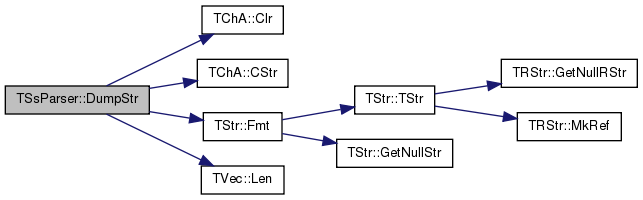bool TSsParser::Eof ( ) const
inline

Checks for end of file.

Definition at line 122 of file ss.h.

Referenced by TSnap::CmtyEvolutionFileBatch(), TTable::GetSchema(), and TSnap::LoadPajek().

122 { return FInPt->Eof(); }
virtual bool Eof()=0
PSIn FInPt
Pointer to the input file stream.
Definition: ss.h:82

Here is the caller graph for this function: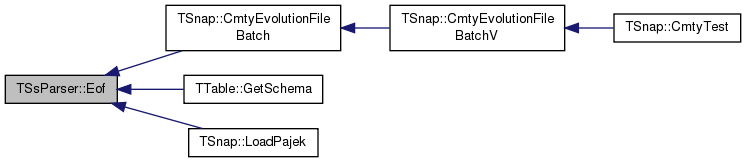const char* TSsParser::GetFld ( const int & FldN ) const
inline

Returns the contents of the field at index `FldN`.

Definition at line 129 of file ss.h.

129 { return FldV[FldN]; }
TVec< char * > FldV
Pointers to fields of the current line.
Definition: ss.h:81

Here is the caller graph for this function: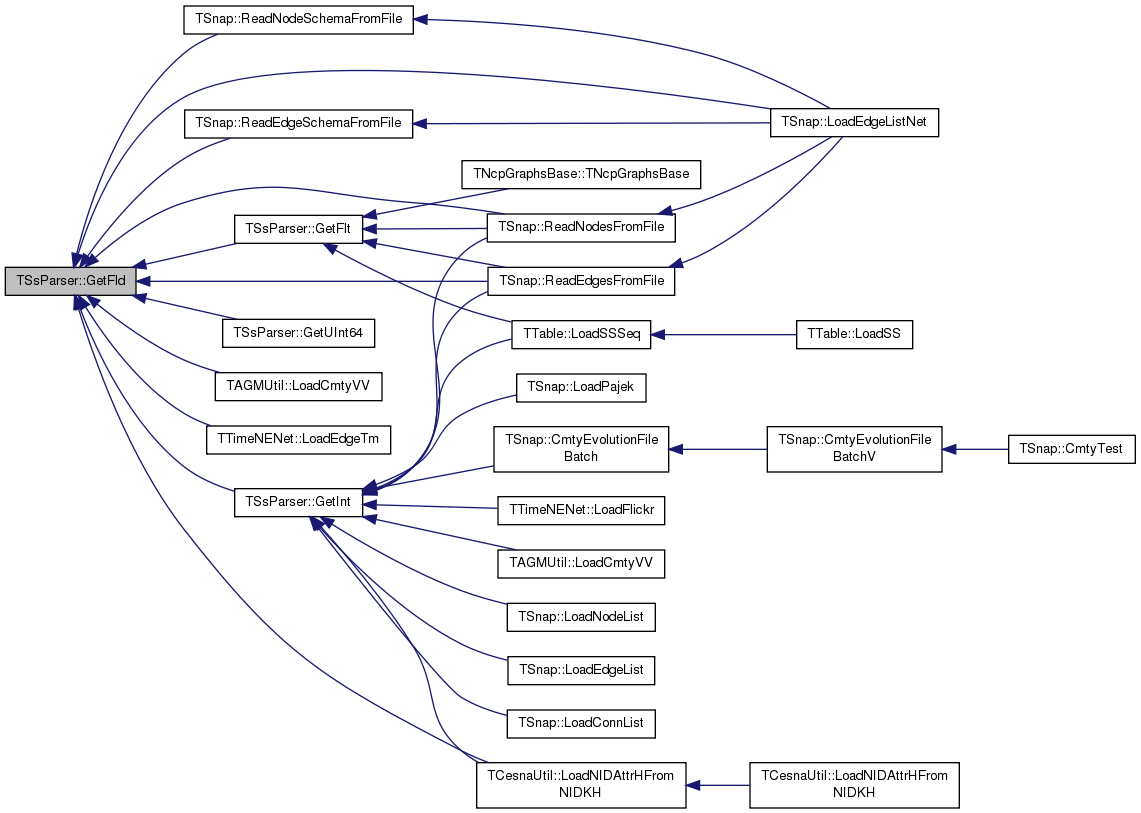char* TSsParser::GetFld ( const int & FldN )
inline

Returns the contents of the field at index `FldN`.

Definition at line 131 of file ss.h.

131 { return FldV[FldN]; }
TVec< char * > FldV
Pointers to fields of the current line.
Definition: ss.h:81
 int TSsParser::GetFlds ( ) const
inline

Returns the number of fields in the current line.

Definition at line 116 of file ss.h.

116 { return Len(); }
int Len() const
Returns the number of fields in the current line.
Definition: ss.h:114

Here is the caller graph for this function: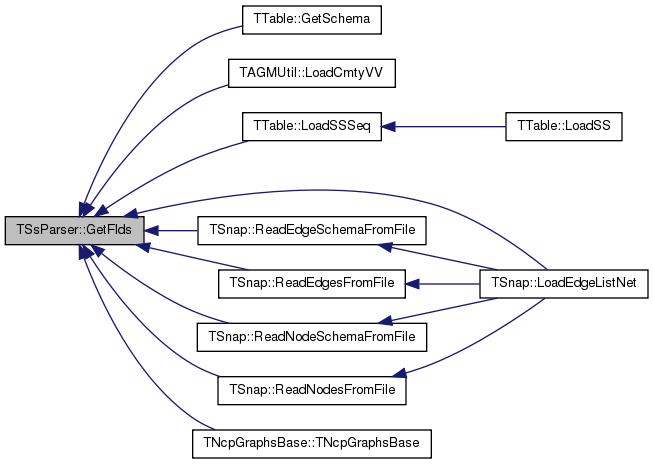bool TSsParser::GetFlt ( const int & FldN, double & Val ) const

If the field `FldN` is a float its value is returned in `Val` and the function returns `true`.

Definition at line 485 of file ss.cpp.

References GetFld(), TCh::IsNum(), TCh::IsWs(), and Len().

485  {
486  // parsing format {ws} [+/-] +{d} ([.]{d}) ([E|e] [+/-] +{d})
487  if (FldN >= Len()) { return false; }
488  const char *c = GetFld(FldN);
489  while (TCh::IsWs(*c)) { c++; }
490  if (*c=='+' || *c=='-') { c++; }
491  if (! TCh::IsNum(*c) && *c!='.') { return false; }
492  while (TCh::IsNum(*c)) { c++; }
493  if (*c == '.') {
494  c++;
495  while (TCh::IsNum(*c)) { c++; }
496  }
497  if (*c=='e' || *c == 'E') {
498  c++;
499  if (*c == '+' || *c == '-' ) { c++; }
500  if (! TCh::IsNum(*c)) { return false; }
501  while (TCh::IsNum(*c)) { c++; }
502  }
503  if (*c != 0) { return false; }
504  Val = atof(GetFld(FldN));
505  return true;
506 }
static bool IsNum(const char &Ch)
Definition: dt.h:1067
const char * GetFld(const int &FldN) const
Returns the contents of the field at index FldN.
Definition: ss.h:129
static bool IsWs(const char &Ch)
Definition: dt.h:1063
int Len() const
Returns the number of fields in the current line.
Definition: ss.h:114

Here is the call graph for this function: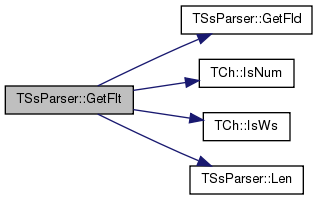Here is the caller graph for this function: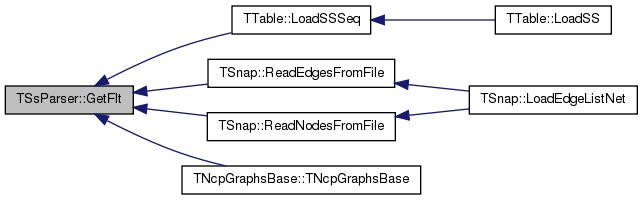double TSsParser::GetFlt ( const int & FldN ) const
inline

Assumes `FldN` is a floating point number its value is returned. If `FldN` is not an integer an exception is thrown.

Definition at line 150 of file ss.h.

References GetFlt(), and IAssert.

Referenced by GetFlt().

150 { double Val=0.0; IAssert(GetFlt(FldN, Val)); return Val; }
#define IAssert(Cond)
Definition: bd.h:262
bool GetFlt(const int &FldN, double &Val) const
If the field FldN is a float its value is returned in Val and the function returns true...
Definition: ss.cpp:485

Here is the call graph for this function: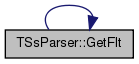Here is the caller graph for this function: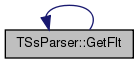bool TSsParser::GetInt ( const int & FldN, int & Val ) const

If the field `FldN` is an integer its value is returned in `Val` and the function returns `true`.

Definition at line 447 of file ss.cpp.

References GetFld(), TCh::GetNum(), TCh::IsNum(), TCh::IsWs(), and Len().

447  {
448  // parsing format {ws} [+/-] +{ddd}
449  if (FldN >= Len()) { return false; }
450  int _Val = -1;
451  bool Minus=false;
452  const char *c = GetFld(FldN);
453  while (TCh::IsWs(*c)) { c++; }
454  if (*c=='-') { Minus=true; c++; }
455  if (! TCh::IsNum(*c)) { return false; }
456  _Val = TCh::GetNum(*c); c++;
457  while (TCh::IsNum(*c)){
458  _Val = 10 * _Val + TCh::GetNum(*c);
459  c++;
460  }
461  if (Minus) { _Val = -_Val; }
462  if (*c != 0) { return false; }
463  Val = _Val;
464  return true;
465 }
static bool IsNum(const char &Ch)
Definition: dt.h:1067
const char * GetFld(const int &FldN) const
Returns the contents of the field at index FldN.
Definition: ss.h:129
static bool IsWs(const char &Ch)
Definition: dt.h:1063
static int GetNum(const char &Ch)
Definition: dt.h:1069
int Len() const
Returns the number of fields in the current line.
Definition: ss.h:114

Here is the call graph for this function: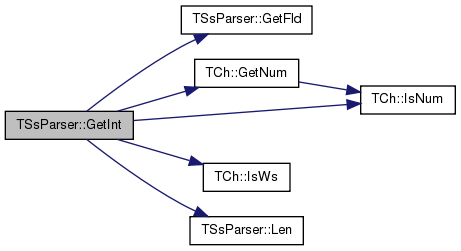Here is the caller graph for this function: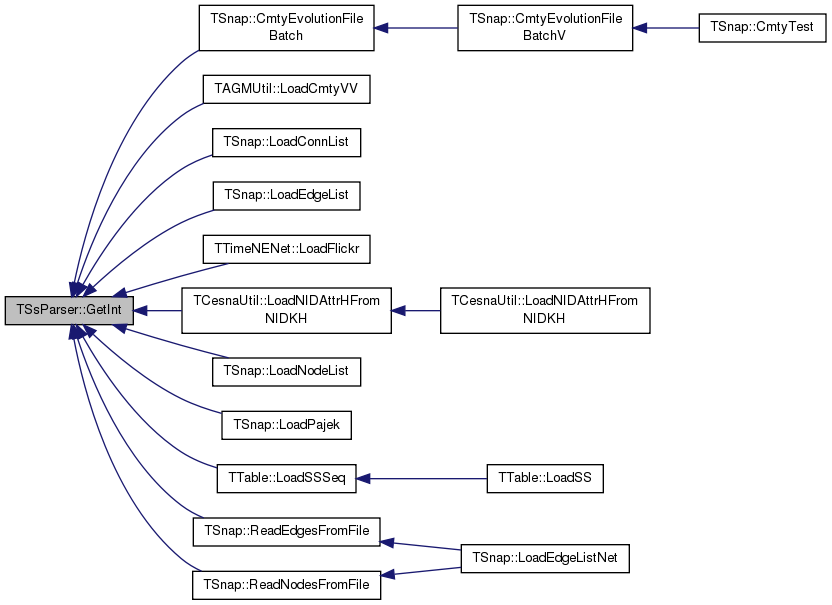int TSsParser::GetInt ( const int & FldN ) const
inline

Assumes `FldN` is an integer its value is returned. If `FldN` is not an integer an exception is thrown.

Definition at line 140 of file ss.h.

References TStr::Fmt(), and IAssertR.

140  {
141  int Val=0; IAssertR(GetInt(FldN, Val), TStr::Fmt("Field %d not INT.\n%s", FldN, DumpStr()).CStr()); return Val; }
#define IAssertR(Cond, Reason)
Definition: bd.h:265
bool GetInt(const int &FldN, int &Val) const
If the field FldN is an integer its value is returned in Val and the function returns true...
Definition: ss.cpp:447
const char * DumpStr() const
Definition: ss.cpp:508
static TStr Fmt(const char *FmtStr,...)
Definition: dt.cpp:1599

Here is the call graph for this function: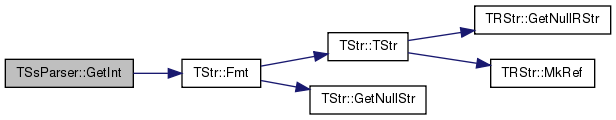uint64 TSsParser::GetLineNo ( ) const
inline

Returns the line number of the current line.

Definition at line 118 of file ss.h.

118 { return LineCnt; }
uint64 LineCnt
Number of processed lines so far.
Definition: ss.h:78

Here is the caller graph for this function: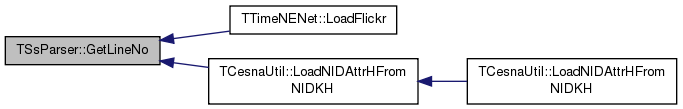TChA TSsParser::GetLnStr ( ) const
inline

Returns the current line.

Definition at line 124 of file ss.h.

References TChA::DelLastCh(), and TChA::Len().

Referenced by TSnap::CmtyEvolutionFileBatch().

124 { TChA LnOut; for (int i = 0; i < Len(); i++) { LnOut+=GetFld(i); LnOut+=' '; } if (LnOut.Len() > 0) LnOut.DelLastCh(); return LnOut; }
int Len() const
Definition: dt.h:259
const char * GetFld(const int &FldN) const
Returns the contents of the field at index FldN.
Definition: ss.h:129
void DelLastCh()
Definition: dt.h:263
int Len() const
Returns the number of fields in the current line.
Definition: ss.h:114
Definition: dt.h:201

Here is the call graph for this function: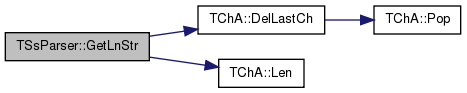Here is the caller graph for this function: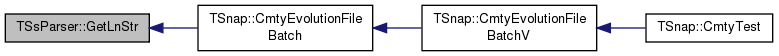bool TSsParser::GetUInt64 ( const int & FldN, uint64 & Val ) const

If the field `FldN` is a 64-bit unsigned integer its value is returned in `Val` and the function returns `true`.

Definition at line 467 of file ss.cpp.

References GetFld(), TCh::GetNum(), TCh::IsNum(), TCh::IsWs(), and Len().

467  {
468  // parsing format {ws} [+]{ddd}
469  if (FldN >= Len()) { return false; }
470  uint64 _Val=0;
471  const char *c = GetFld(FldN);
472  while (TCh::IsWs(*c)){ c++; }
473  if (*c == '+'){ c++; }
474  if (! TCh::IsNum(*c)) { return false; }
475  _Val = TCh::GetNum(*c); c++;
476  while (TCh::IsNum(*c)) {
477  _Val = 10*_Val + TCh::GetNum(*c);
478  c++;
479  }
480  if (*c != 0) { return false; }
481  Val = _Val;
482  return true;
483 }
static bool IsNum(const char &Ch)
Definition: dt.h:1067
const char * GetFld(const int &FldN) const
Returns the contents of the field at index FldN.
Definition: ss.h:129
static bool IsWs(const char &Ch)
Definition: dt.h:1063
unsigned long long uint64
Definition: bd.h:38
static int GetNum(const char &Ch)
Definition: dt.h:1069
int Len() const
Returns the number of fields in the current line.
Definition: ss.h:114

Here is the call graph for this function: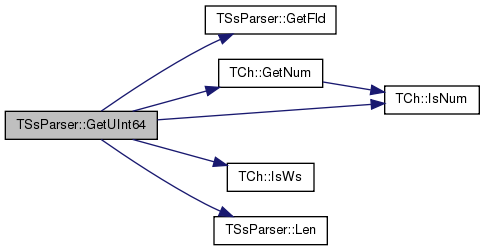uint64 TSsParser::GetUInt64 ( const int & FldN ) const
inline

Assumes `FldN` is a 64-bit unsigned integer point number its value is returned. If `FldN` is not a 64-bit unsigned integer an exception is thrown.

Definition at line 157 of file ss.h.

References GetUInt64(), and IAssert.

Referenced by GetUInt64().

157 { uint64 Val=0; IAssert(GetUInt64(FldN, Val)); return Val; }
#define IAssert(Cond)
Definition: bd.h:262
bool GetUInt64(const int &FldN, uint64 &Val) const
If the field FldN is a 64-bit unsigned integer its value is returned in Val and the function returns ...
Definition: ss.cpp:467
unsigned long long uint64
Definition: bd.h:38

Here is the call graph for this function: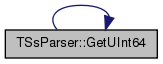Here is the caller graph for this function: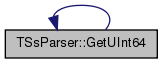bool TSsParser::IsCmt ( ) const
inline

Checks whether the current line is a comment (starts with '#').

Definition at line 120 of file ss.h.

120 { return Len()>0 && GetFld(0) == '#'; }
const char * GetFld(const int &FldN) const
Returns the contents of the field at index FldN.
Definition: ss.h:129
int Len() const
Returns the number of fields in the current line.
Definition: ss.h:114

Here is the caller graph for this function: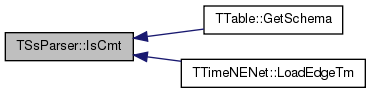bool TSsParser::IsFlt ( const int & FldN ) const
inline

Checks whether fields `FldN` is a float.

Definition at line 148 of file ss.h.

Referenced by TTable::GetSchema(), and TNcpGraphsBase::TNcpGraphsBase().

148 { double v; return GetFlt(FldN, v); }
bool GetFlt(const int &FldN, double &Val) const
If the field FldN is a float its value is returned in Val and the function returns true...
Definition: ss.cpp:485

Here is the caller graph for this function: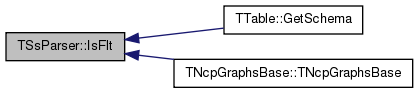bool TSsParser::IsInt ( const int & FldN ) const
inline

Checks whether fields `FldN` is an integer.

Definition at line 143 of file ss.h.

143 { int v; return GetInt(FldN, v); }
bool GetInt(const int &FldN, int &Val) const
If the field FldN is an integer its value is returned in Val and the function returns true...
Definition: ss.cpp:447

Here is the caller graph for this function: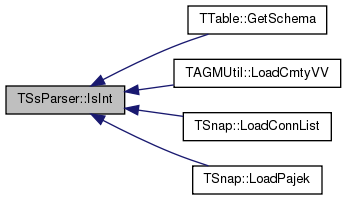bool TSsParser::IsUInt64 ( const int & FldN ) const
inline

Checks whether fields `FldN` is unsigned 64-bit integer number.

Definition at line 155 of file ss.h.

155 { uint64 v; return GetUInt64(FldN, v); }
bool GetUInt64(const int &FldN, uint64 &Val) const
If the field FldN is a 64-bit unsigned integer its value is returned in Val and the function returns ...
Definition: ss.cpp:467
unsigned long long uint64
Definition: bd.h:38
 int TSsParser::Len ( ) const
inline

Returns the number of fields in the current line.

Definition at line 114 of file ss.h.

114 { return FldV.Len(); }
TSizeTy Len() const
Returns the number of elements in the vector.
Definition: ds.h:575
TVec< char * > FldV
Pointers to fields of the current line.
Definition: ss.h:81

Here is the caller graph for this function: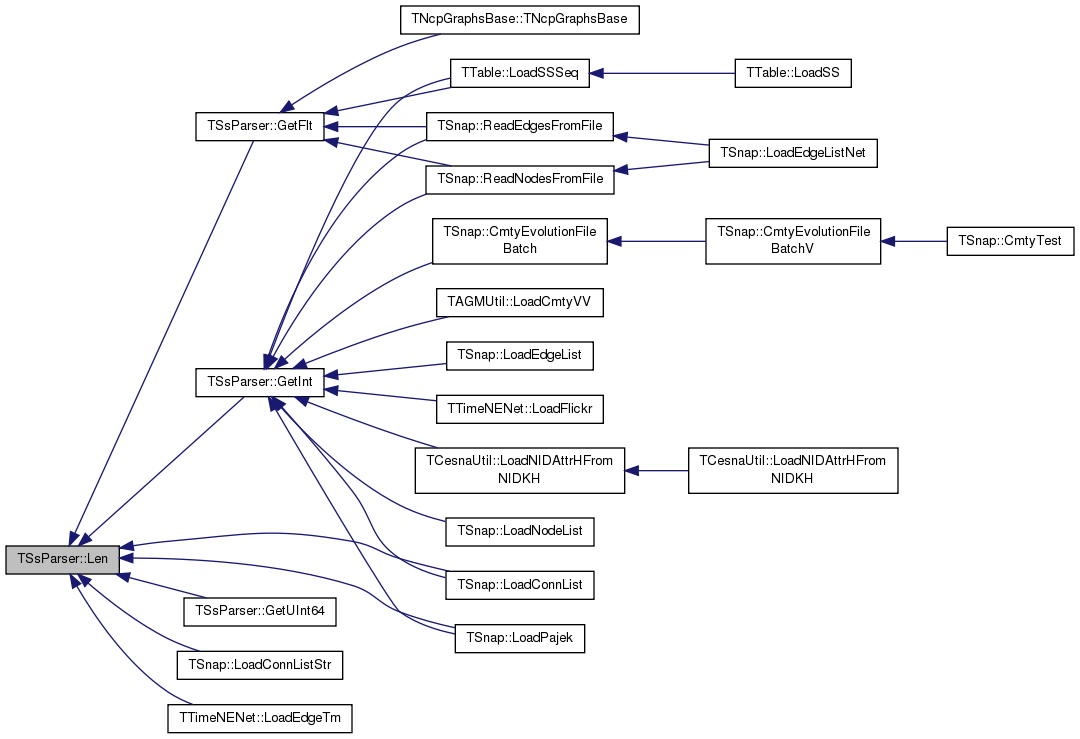static PSsParser TSsParser::New ( const TStr & FNm, const TSsFmt SsFmt )
inlinestatic

Definition at line 102 of file ss.h.

102 { return new TSsParser(FNm, SsFmt); }
TSsFmt SsFmt
Separator type.
Definition: ss.h:74
TSsParser(const TStr &FNm, const TSsFmt _SsFmt=ssfTabSep, const bool &_SkipLeadBlanks=false, const bool &_SkipCmt=true, const bool &_SkipEmptyFld=false)
Constructor.
Definition: ss.cpp:351
 bool TSsParser::Next ( )

Loads next line from the input file.

If end of file is reached, return value is `false`.

Definition at line 412 of file ss.cpp.

412  { // split on SplitCh
413  FldV.Clr(false);
414  LineStr.Clr();
415  FldV.Clr();
416  LineCnt++;
417  if (! FInPt->GetNextLnBf(LineStr)) { return false; }
418  if (SkipCmt && !LineStr.Empty() && LineStr=='#') { return Next(); }
419
420  char* cur = LineStr.CStr();
422  while (*cur && TCh::IsWs(*cur)) { cur++; }
423  }
424  char *last = cur;
425  while (*cur) {
426  if (SsFmt == ssfWhiteSep) { while (*cur && ! TCh::IsWs(*cur)) { cur++; } }
427  else { while (*cur && *cur!=SplitCh) { cur++; } }
428  if (*cur == 0) { break; }
429  *cur = 0; cur++;
431  if (SkipEmptyFld && strlen(FldV.Last())==0) { FldV.DelLast(); } // skip empty fields
432  }
433
434  if (*last != 0) { FldV.Add(last); } // add last field
435  if (SkipEmptyFld && FldV.Empty()) { return Next(); } // skip empty lines
436
437  return true;
438 }
TSsFmt SsFmt
Separator type.
Definition: ss.h:74
bool Empty() const
Definition: dt.h:260
void Clr()
Definition: dt.h:258
uint64 LineCnt
Number of processed lines so far.
Definition: ss.h:78
bool Empty() const
Tests whether the vector is empty.
Definition: ds.h:570
TVec< char * > FldV
Pointers to fields of the current line.
Definition: ss.h:81
static bool IsWs(const char &Ch)
Definition: dt.h:1063
TChA LineStr
Current line.
Definition: ss.h:80
void Clr(const bool &DoDel=true, const TSizeTy &NoDelLim=-1)
Clears the contents of the vector.
Definition: ds.h:1022
char * CStr()
Definition: dt.h:255
Whitespace (space or tab) separated.
Definition: ss.h:11
const TVal & Last() const
Returns a reference to the last element of the vector.
Definition: ds.h:579
PSIn FInPt
Pointer to the input file stream.
Definition: ss.h:82
bool SkipCmt
Skip comments (lines starting with #).
Definition: ss.h:76
Ignore leading whitespace characters in a line.
Definition: ss.h:75
bool Next()
Loads next line from the input file.
Definition: ss.cpp:412
char SplitCh
Separator character (if one of the non-started separators is used)
Definition: ss.h:79
virtual bool GetNextLnBf(TChA &LnChA)=0
Adds a new element at the end of the vector, after its current last element.
Definition: ds.h:602
void DelLast()
Removes the last element of the vector.
Definition: ds.h:665
bool SkipEmptyFld
Skip empty fields (i.e., multiple consecutive separators are considered as one).
Definition: ss.h:77

Here is the call graph for this function: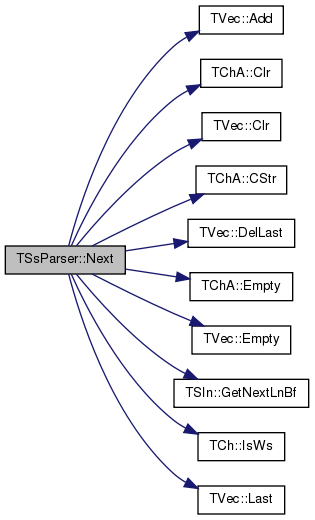Here is the caller graph for this function: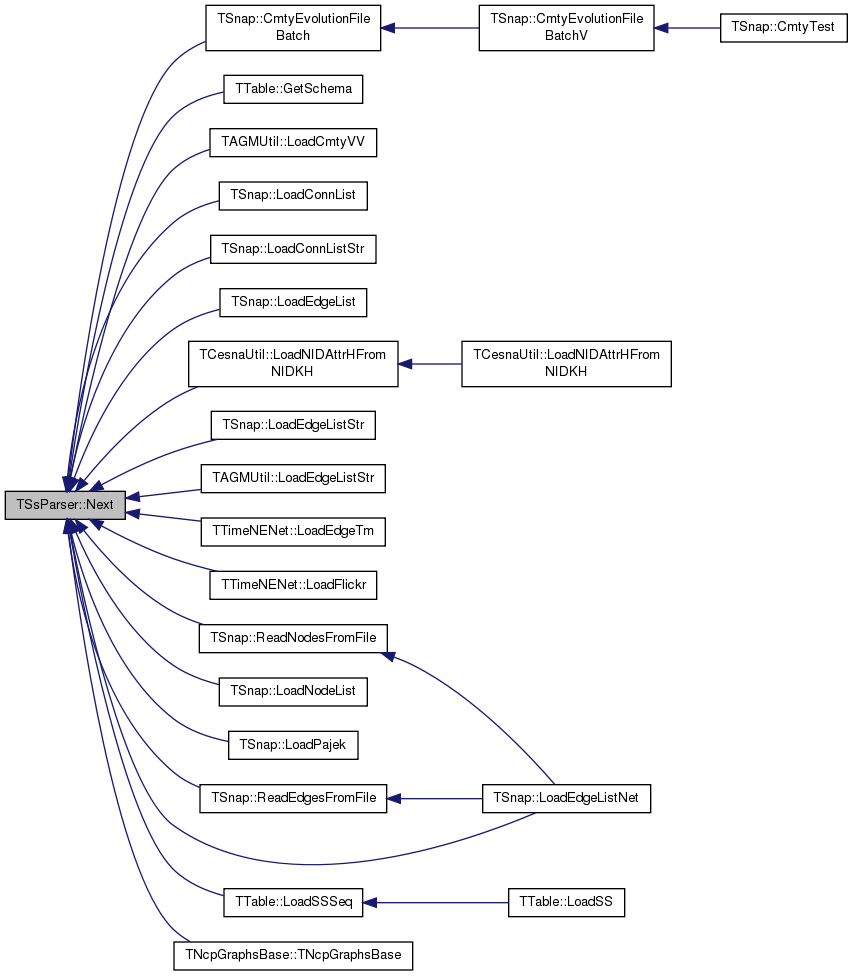bool TSsParser::NextSlow ( )

Loads next line from the input file (older, slow implementation - deprecated).

If end of file is reached, return value is `false`. This function is deprecated, use Next instead.

Definition at line 382 of file ss.cpp.

382  { // split on SplitCh
383  FldV.Clr(false);
384  LineStr.Clr();
385  FldV.Clr();
386  LineCnt++;
387  if (! FInPt->GetNextLn(LineStr)) { return false; }
388  if (SkipCmt && !LineStr.Empty() && LineStr=='#') { return NextSlow(); }
389
390  char* cur = LineStr.CStr();
392  while (*cur && TCh::IsWs(*cur)) { cur++; }
393  }
394  char *last = cur;
395  while (*cur) {
396  if (SsFmt == ssfWhiteSep) { while (*cur && ! TCh::IsWs(*cur)) { cur++; } }
397  else { while (*cur && *cur!=SplitCh) { cur++; } }
398  if (*cur == 0) { break; }
399  *cur = 0; cur++;
401  if (SkipEmptyFld && strlen(FldV.Last())==0) { FldV.DelLast(); } // skip empty fields
402  }
403
404  if (*last != 0) { FldV.Add(last); } // add last field
405  if (SkipEmptyFld && FldV.Empty()) { return NextSlow(); } // skip empty lines
406
407  return true;
408 }
TSsFmt SsFmt
Separator type.
Definition: ss.h:74
bool Empty() const
Definition: dt.h:260
void Clr()
Definition: dt.h:258
bool NextSlow()
Loads next line from the input file (older, slow implementation - deprecated).
Definition: ss.cpp:382
uint64 LineCnt
Number of processed lines so far.
Definition: ss.h:78
bool Empty() const
Tests whether the vector is empty.
Definition: ds.h:570
TVec< char * > FldV
Pointers to fields of the current line.
Definition: ss.h:81
static bool IsWs(const char &Ch)
Definition: dt.h:1063
TChA LineStr
Current line.
Definition: ss.h:80
void Clr(const bool &DoDel=true, const TSizeTy &NoDelLim=-1)
Clears the contents of the vector.
Definition: ds.h:1022
char * CStr()
Definition: dt.h:255
Whitespace (space or tab) separated.
Definition: ss.h:11
const TVal & Last() const
Returns a reference to the last element of the vector.
Definition: ds.h:579
PSIn FInPt
Pointer to the input file stream.
Definition: ss.h:82
bool SkipCmt
Skip comments (lines starting with #).
Definition: ss.h:76
Ignore leading whitespace characters in a line.
Definition: ss.h:75
char SplitCh
Separator character (if one of the non-started separators is used)
Definition: ss.h:79
Adds a new element at the end of the vector, after its current last element.
Definition: ds.h:602
void DelLast()
Removes the last element of the vector.
Definition: ds.h:665
bool SkipEmptyFld
Skip empty fields (i.e., multiple consecutive separators are considered as one).
Definition: ss.h:77
bool GetNextLn(TStr &LnStr)
Definition: fl.cpp:43

Here is the call graph for this function: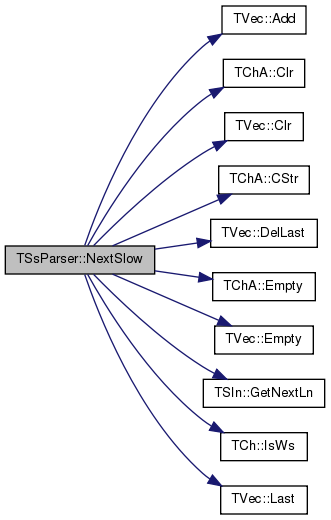const char* TSsParser::operator[] ( const int & FldN ) const
inline

Returns the contents of the field at index `FldN`.

Definition at line 133 of file ss.h.

133 { return FldV[FldN]; }
TVec< char * > FldV
Pointers to fields of the current line.
Definition: ss.h:81
 char* TSsParser::operator[] ( const int & FldN )
inline

Returns the contents of the field at index `FldN`.

Definition at line 135 of file ss.h.

135 { return FldV[FldN]; }
TVec< char * > FldV
Pointers to fields of the current line.
Definition: ss.h:81
 void TSsParser::ToLc ( )

Transforms the current line to lower case.

Definition at line 440 of file ss.cpp.

References FldV, and TVec< TVal, TSizeTy >::Len().

440  {
441  for (int f = 0; f < FldV.Len(); f++) {
442  for (char *c = FldV[f]; *c; c++) {
443  *c = tolower(*c); }
444  }
445 }
TSizeTy Len() const
Returns the number of elements in the vector.
Definition: ds.h:575
TVec< char * > FldV
Pointers to fields of the current line.
Definition: ss.h:81

Here is the call graph for this function: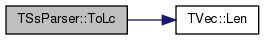Here is the caller graph for this function: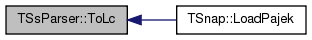TSsParser::UndefDefaultCopyAssign ( TSsParser )
private

## Friends And Related Function Documentation

 friend class TPt< TSsParser >
friend

Definition at line 72 of file ss.h.

## Member Data Documentation

 TCRef TSsParser::CRef
private

Definition at line 72 of file ss.h.

 PSIn TSsParser::FInPt
private

Pointer to the input file stream.

Definition at line 82 of file ss.h.

Referenced by Next(), NextSlow(), and TSsParser().

 TVec TSsParser::FldV
private

Pointers to fields of the current line.

Definition at line 81 of file ss.h.

Referenced by DumpStr(), Next(), NextSlow(), and ToLc().

 uint64 TSsParser::LineCnt
private

Number of processed lines so far.

Definition at line 78 of file ss.h.

Referenced by Next(), and NextSlow().

 TChA TSsParser::LineStr
private

Current line.

Definition at line 80 of file ss.h.

Referenced by Next(), and NextSlow().

 bool TSsParser::SkipCmt
private

Skip comments (lines starting with #).

Definition at line 76 of file ss.h.

Referenced by Next(), and NextSlow().

 bool TSsParser::SkipEmptyFld
private

Skip empty fields (i.e., multiple consecutive separators are considered as one).

Definition at line 77 of file ss.h.

Referenced by Next(), and NextSlow().

private

Ignore leading whitespace characters in a line.

Definition at line 75 of file ss.h.

Referenced by Next(), and NextSlow().

 char TSsParser::SplitCh
private

Separator character (if one of the non-started separators is used)

Definition at line 79 of file ss.h.

Referenced by Next(), NextSlow(), and TSsParser().

 TSsFmt TSsParser::SsFmt
private

Separator type.

Definition at line 74 of file ss.h.

Referenced by Next(), NextSlow(), and TSsParser().

The documentation for this class was generated from the following files: